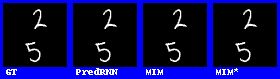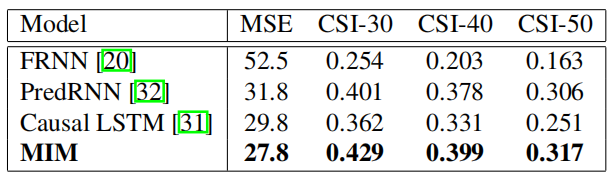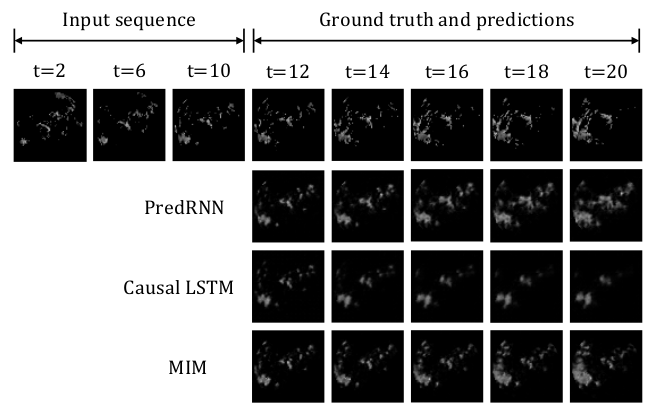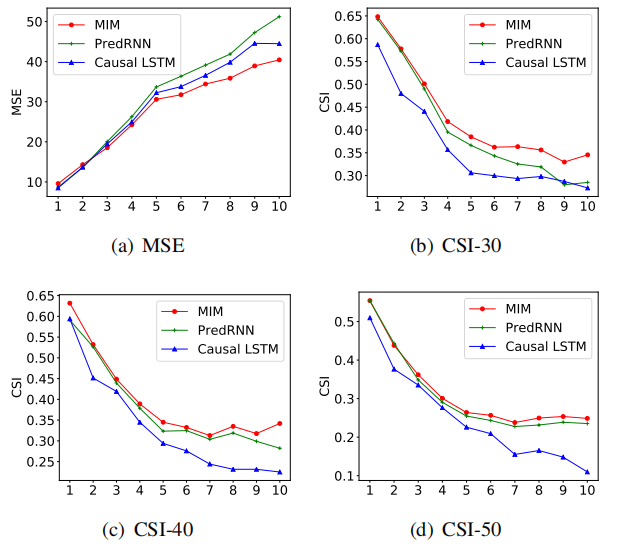## ICCV 2019

### CVP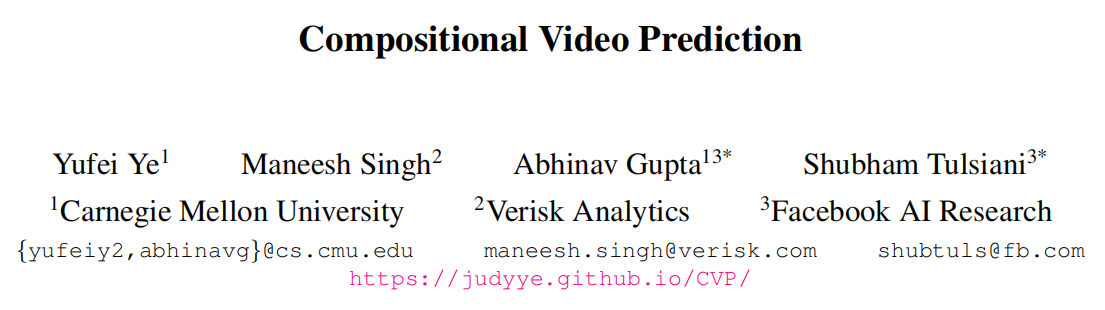github地址：https://github.com/JudyYe/CVP

​ 该论文使用了两个数据集，一个是包含可能掉落的堆叠物体ShapeStacks，另一个包含人类在体育馆中进行活动的视频Penn Action，并表明论文的方法可以在这些不同的环境中进行逼真的随机视频预测。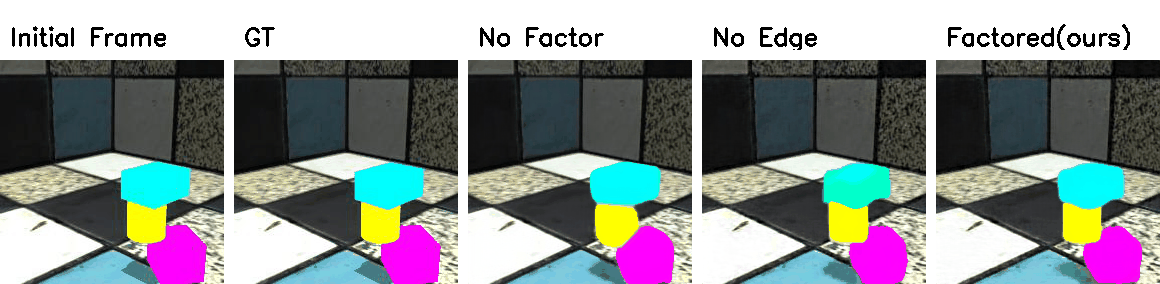​ 主要架构有下面三个部分组成：

1. Entity Predictor（实体预测模块）: 预测每一个实体表示的未来状态

2. Frame Decoder（帧解码器）: 从实体表示中解码成frame

3. Encoder（编码器）: 把frame编码成u作为LSTM的cell-state得到输出记录时序信息$$z^1...z^t$$

（最后其实就是concat进去，见如下:：）

obj_vecs = torch.cat([pose, bbox, diff_z], dim=-1)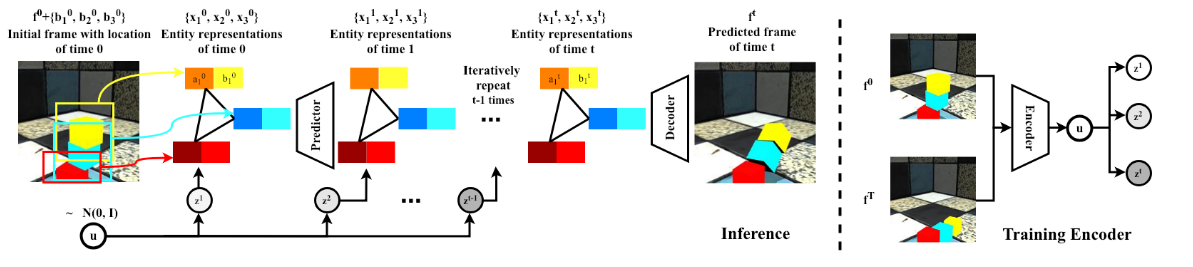$$\left\{\left(\bar{\phi}_{n}, \bar{M}_{n}\right)=g\left(a_{n}\right)\right\}_{n=1}^{N}$$表示在g的网络结构下解码每一个实体的特征和空间掩码，让$$\mathcal{W}$$表示类似Spatial Transformer Networks的空间变化网络，可以得到下面的实体的特征和掩码$$\phi_{n}$$$$M_n$$.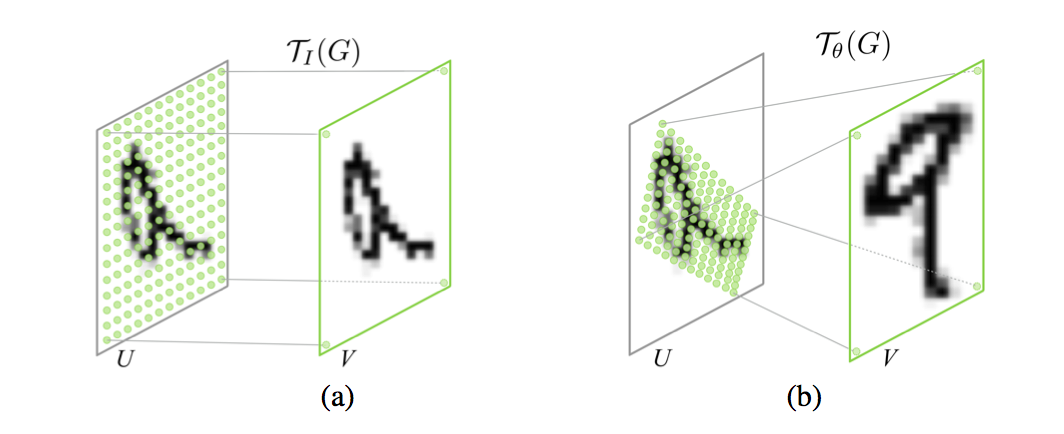$\phi_{n}=\mathcal{W}\left(\bar{\phi}_{n}, b_{n}\right) ; \quad M_{n}=\mathcal{W}\left(\bar{M}_{n}, b_{n}\right)$

$\phi=\frac{\phi_{b g} \odot M_{b g} {\oplus \sum_{n} \phi_{n} \odot M_{n}}}{M_{b g}\oplus\sum_{n} M_{n}}$

$L_{e n c}=K L[q(u) \| \mathcal{N}(0, I)]$

$L_{d e c}=\sum_{t=0}^{T}\left\|\mathcal{D}\left(\left\{\hat{x}_{n}^{t}\right\}, f^{0}\right)-\hat{f}^{t}\right\|_{1}$

$L_{p r e d}=\sum_{t=1}^{T}\left(\left\|\mathcal{D}\left(\left\{x_{n}^{t}\right\}, f^{0}\right)-\hat{f}^{t}\right\|_{1}+\lambda_{1} \sum_{n=1}^{N}\left\|b_{n}^{t}-\hat{b}_{n}^{t}\right\|^{2}\right)$

$L=L_{d e c}+L_{p r e d}+\lambda_{2} L_{e n c}$

### Non-Local ConvLSTM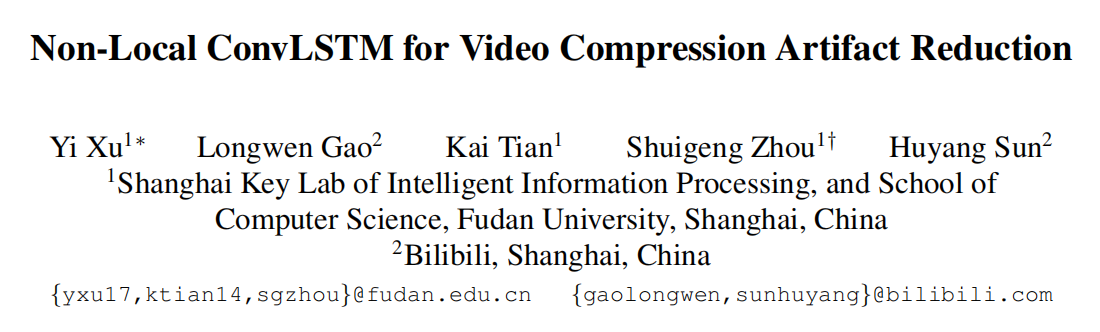github地址：https://github.com/xyiyy/NL-ConvLSTM (Code will be published later)

Non-Local ConvLSTM是复旦大学和b站的论文，其实这篇不太算视频预测方向，这是使用了在ConvLSTM中使用Non-Local结合前一帧增强图像减少视频压缩的伪影，是视频压缩的领域，但是对我有些启发，Non-Local最初就是用于视频分类的。

SSIM是用来评价整张图的质量，但是对于一张质量不好的图来说他的patch并不一定差，对于一张好图来说他的patch也不一定好，所以作者用Non-Local来捕捉两帧之间特征图间像素的相似性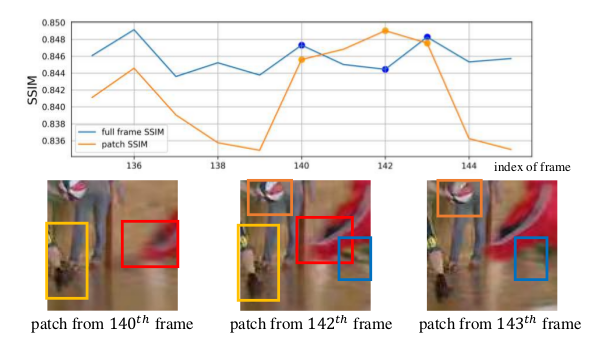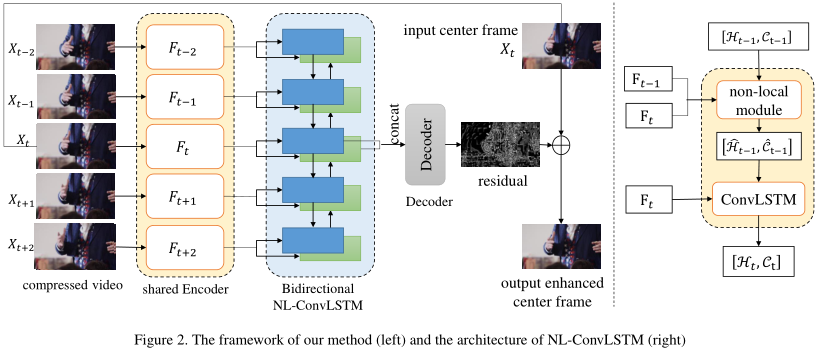ConvLSTM可以表示成下面的公式：
$\left[\mathcal{H}_{t}, \mathcal{C}_{t}\right]=\operatorname{Conv} L S T M\left(F_{t},\left[\mathcal{H}_{t-1}, \mathcal{C}_{t-1}\right]\right)$
hidden state ($$H_t,C_t$$) 是从上一次的hidden state ($$H_{t_1},C_{t-1}$$) 和$$F_t$$ 经过ConvLSTM-cell得到的。

NL-ConvLSTM是在ConvLSTM的基础上加了Non-local的方法，可以表示如下：
\begin{aligned} S_{t} &=N L\left(F_{t-1}, F_{t}\right) \\\left[\hat{\mathcal{H}}_{t-1}, \hat{\mathcal{C}}_{t-1}\right] &=N L W \operatorname{arp}\left(\left[\mathcal{H}_{t-1}, \mathcal{C}_{t-1}\right], S_{t}\right) \\\left[H_{t}, C_{t}\right] &=\operatorname{Conv} L S T M\left(F_{t},\left[\hat{\mathcal{H}}_{t-1}, \hat{c}_{t-1}\right]\right) \end{aligned}
​ 其中$$S_{t} \in \mathbb{R}^{N \times N}$$是当前帧$$F_t$$ 与前一帧的$$F_{t-1}$$ 的相似矩阵，这里的Non-Local的操作是一种特殊的attention，这不是self-attention，是比较前一帧获得相似矩阵再计算attentionNLWrap操作可以用数学表达如下：
\begin{aligned} D_{t}(i, j) &=\left\|F_{t-1}(i)-F_{t}(j)\right\|_{2} \\ S_{t}(i, j) &=\frac{\exp \left(-D_{t}(i, j) / \beta\right)}{\sum_{\forall i} \exp \left(-D_{t}(i, j) / \beta\right)} \\\left[\hat{\mathcal{H}}_{t-1}, \hat{\mathcal{C}}_{t-1}\right] &=\left[\mathcal{H}_{t} \cdot S_{t}, \mathcal{C}_{t} \cdot S_{t}\right] \end{aligned}

$\left[\hat{\mathcal{H}}_{t-1}, \hat{\mathcal{C}}_{t-1}\right] =\left[\mathcal{H}_{t-1} \cdot S_{t}, \mathcal{C}_{t-1} \cdot S_{t}\right]$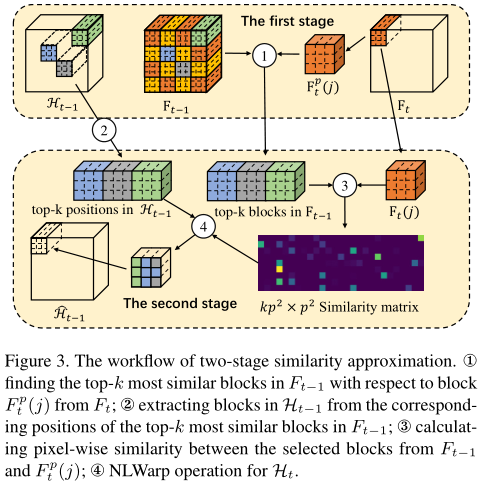## ICLR 2019

### SAVP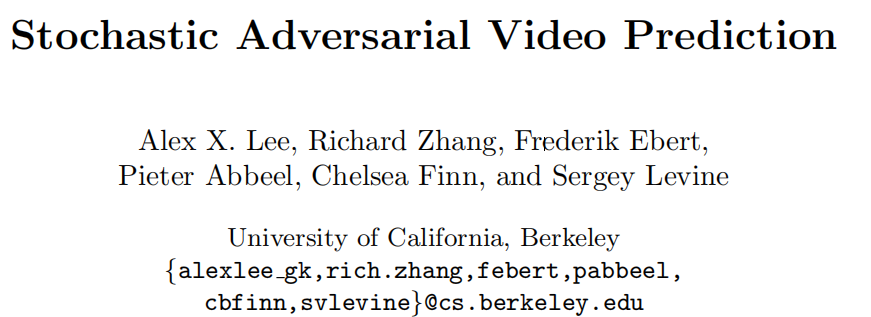​ 当我们与环境中的对象进行交互时，我们可以轻松地想象我们的行为所产生的后果：推一颗球，它会滚走；扔一个花瓶，它会碎掉。视频预测中的主要挑战是问题的模棱两可，未来的发展方向似乎有太多。就像函数的导数能够预测该值附近的走向，当我们预测非常接近的未来时我们能够未来可期，可是当可能性的空间超出了几帧之后，并且该问题本质上变成了多模的，即预测就变得更多样了。​ 这篇把GAN和VAE都用在了视频预测里，其实GAN-VAE在生成方面早有人结合，只是在视频预测中没有人提出，其实提出的SAVP是SV2P (Stochastic Variational Video Prediction) 和SVG-LP (Stochastic Video Generation with a Learned Prior) 的结合。

SV2P网络结构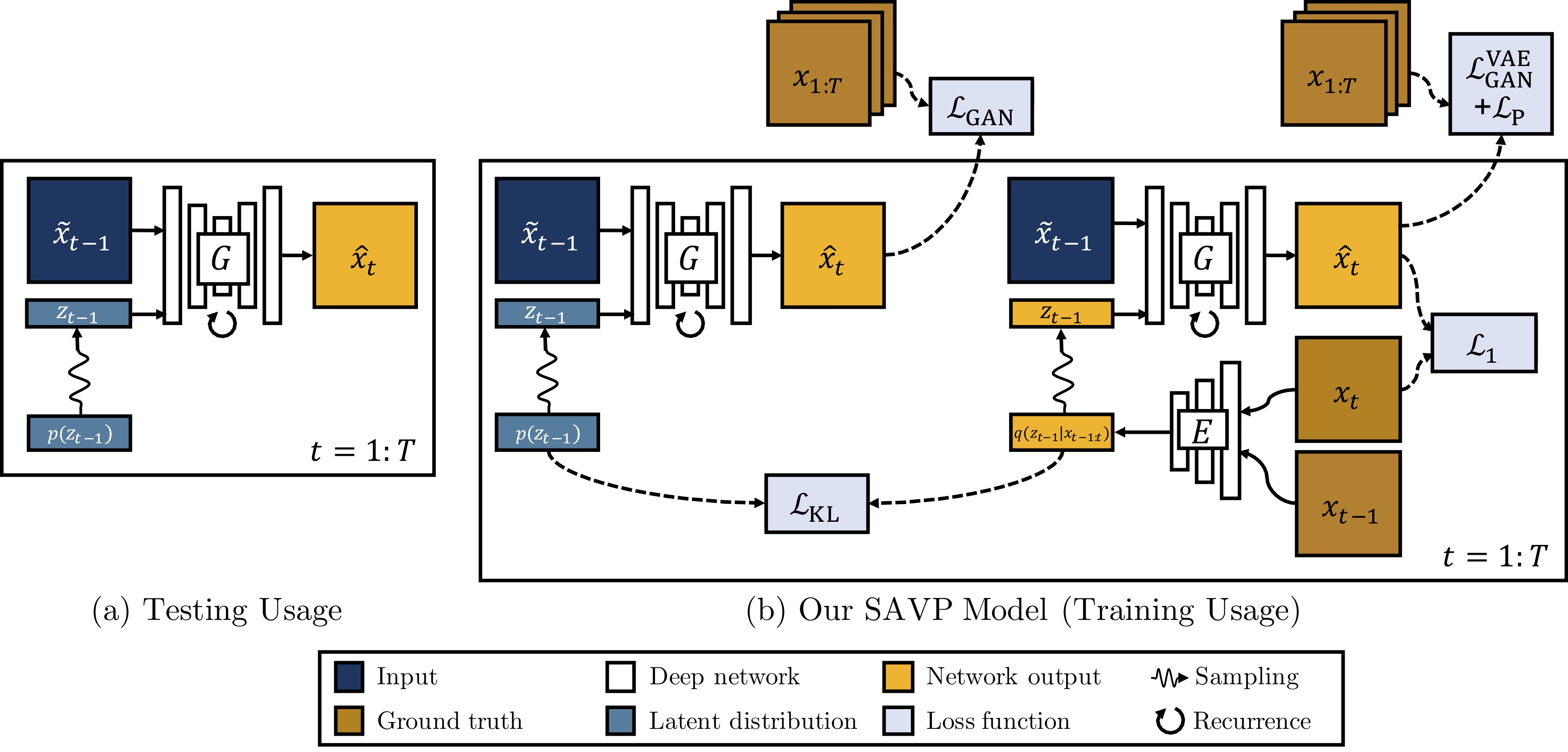​ 在训练期间，隐变量从$$q(z_{t-1}|x_{t-1:t})$$中采样，对每一帧的生成都可以看作是对$$\hat{x}_{t}$$的重构，$$x_t$$$${x}_{t-1}$$被Encoder编码为隐变量$$z_{t-1}$$，前一帧$$x_{t-1}$$与隐变量$$z_{t-1}$$经过G模型之后得到预测帧$$\hat{x}_t$$要计算与当前帧$$x_t$$$$L_1$$损失，使其生成要尽量相似。
$\mathcal{L}_{1}(G, E)=\mathbb{E}_{\mathbf{x}_{0: T}\left.\mathbf{z}_{t} \sim E\left(\mathbf{x}_{t: t+1}\right)\right|_{t=0} ^{T-1}}{\left[\sum_{t=1}^{T}\left\|\mathbf{x}_{t}-G\left(\mathbf{x}_{t-1}, \mathbf{z}_{t-1}\right)\right\|_{1}\right]}.$
​ 在测试阶段我们的隐变量从先验分布$$p(z_{t-1})$$直接采样，$$z_{t-1}$$$$\tilde{x}_{t-1}$$ 经过G生成下一帧的预测图$$\hat{x}_t$$ ，所以需要同时拉近$$q(z_{t-1}|x_{t-1:t})$$$$p(z_{t-1})$$的分布，其KL散度如下：
$\mathcal{L}_{\mathrm{KL}}(E)=\mathbb{E}_{\mathbf{x}_{0: T}}\left[\sum_{t=1}^{T} \mathcal{D}_{\mathrm{KL}}\left(E\left(\mathbf{x}_{t-1: t}\right) \| p\left(\mathbf{z}_{t-1}\right)\right)\right]$
​ 所以G和E的目标函数如下：
$G^{*}, E^{*}=\arg \min _{G, E} \lambda_{1} \mathcal{L}_{1}(G, E)+\lambda_{\mathrm{KL}} \mathcal{L}_{\mathrm{KL}}(E)$
$$L_1$$损失并不是很能反映图像的相似度，既然文章是VAE和GAN的结合，所以在下面提出了判别器去评判图片质量。论文指出单纯的VAE更容易产生模糊图，这里加入的判别器是能够分辨出生成视频序列$$\hat{x}_{1:T}$$与真实视频序列$$x_{1:T}$$，这里是比较意想不到的地方，因为这里没有使用直接的图像判别器，而是判别生成序列与真实序列，其D判别器的网络结构是使用了3D卷积基于SNGAN的，G生成器是使用了convLSTM捕捉时空序列信息。
$\mathcal{L}_{\mathrm{GAN}}(G, D)=\mathbb{E}_{\mathbf{x}_{1: T}}\left[\log D\left(\mathbf{x}_{1: T}\right)\right]+\mathbb{E}_{\left.\mathbf{x}_{1: T}, \mathbf{z}_{t} \sim p\left(\mathbf{z}_{t}\right)\right)_{t=0}^{T-1}}\left[\log \left(1-D\left(G\left(\mathbf{x}_{0}, \mathbf{z}_{0: T-1}\right)\right)\right)\right]$
​ 最后总的损失函数如下：
$G^{*}, E^{*}=\arg \min _{G, E} \max _{D, D^{\mathrm{ME}}} \lambda_{1} \mathcal{L}_{1}(G, E)+\lambda_{\mathrm{KL}} \mathcal{L}_{\mathrm{KL}}(E)+\mathcal{L}_{\mathrm{GAN}}(G, D)+\mathcal{L}_{\mathrm{GAN}}^{\mathrm{VAE}}\left(G, E, D^{\mathrm{VAE}}\right)$
​ 下面是论文中的实验结果：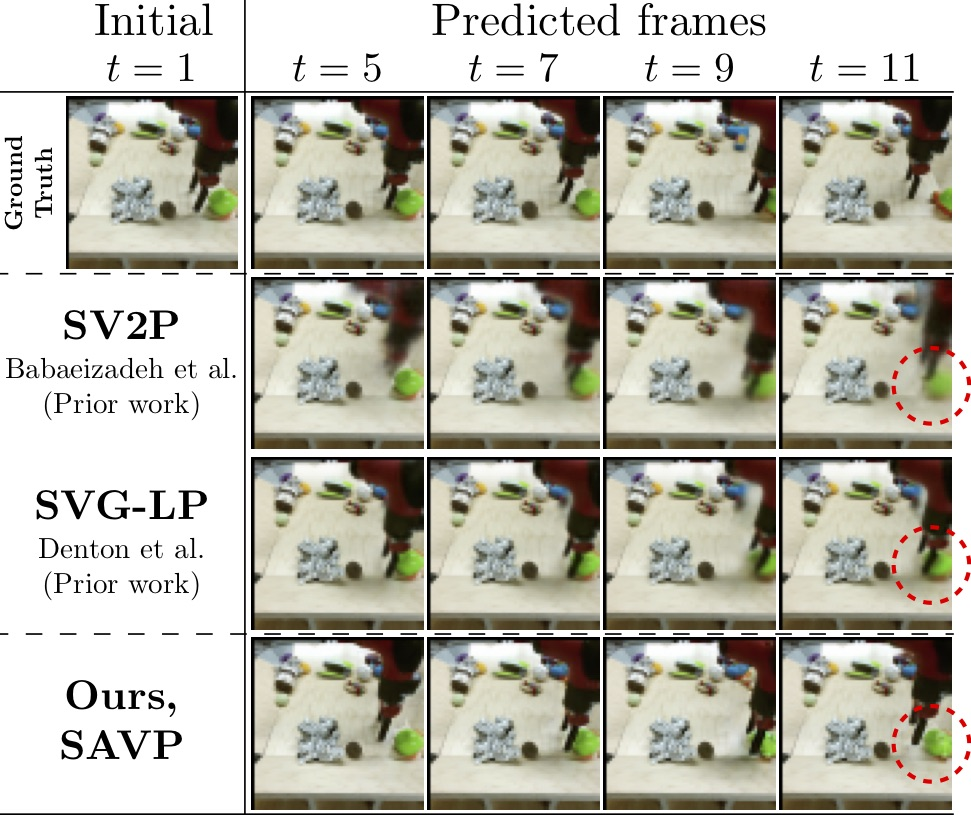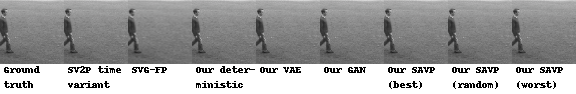## CVPR 2019

### MIM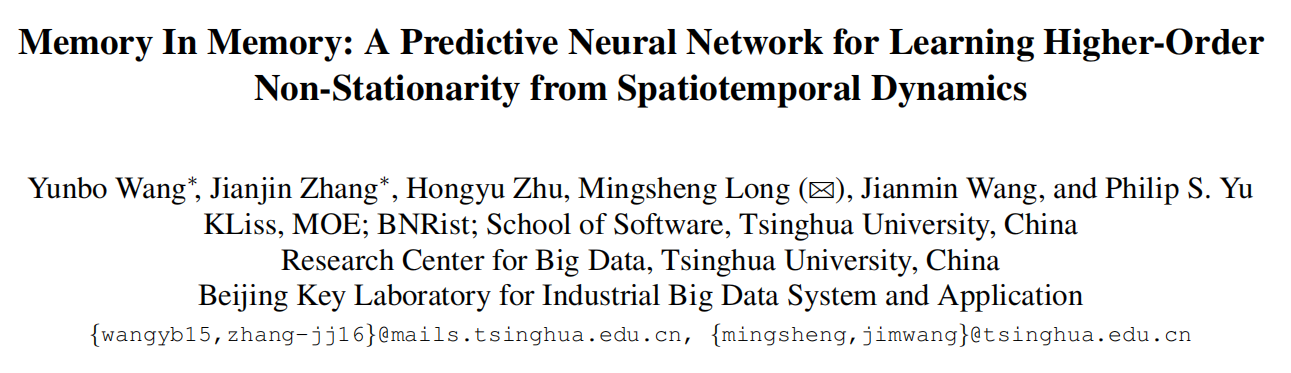github地址：https://github.com/Yunbo426/MIM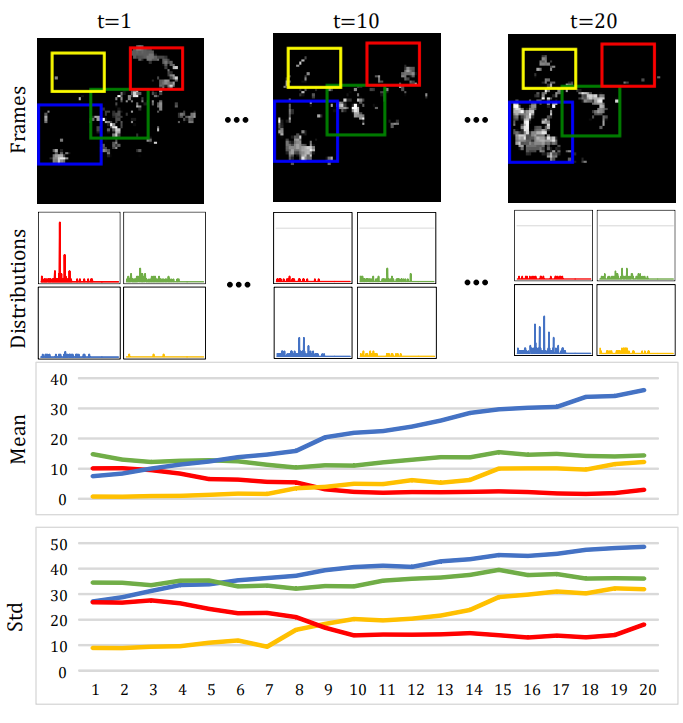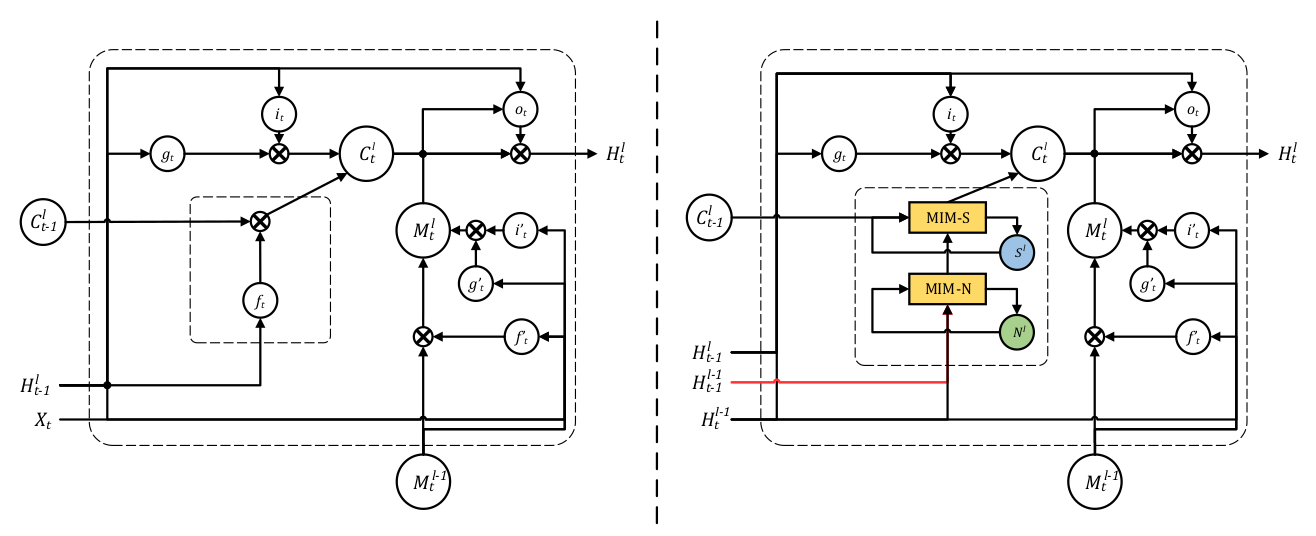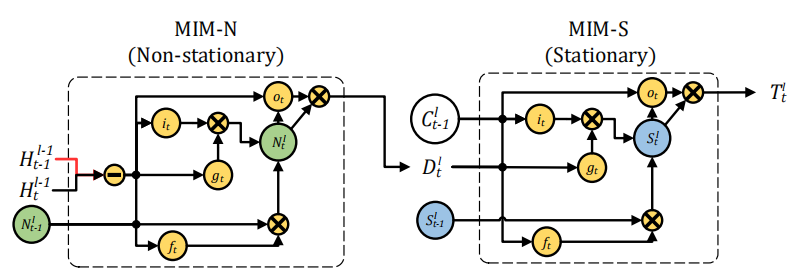MIM-N所有的门$$g_t$$$$i_t$$$$f_t$$ ，和$$o_t$$都用$$\left(\mathcal{H}_{t}^{l-1}-\mathcal{H}_{t-1}^{l-1}\right)$$ 短期记忆的隐状态的帧差更新，因为这样强调了非平稳变换，最后得到特征差$$D_t^l$$$$C^l_{t-1}$$作为MIM-S输入，MIM-S会根据原记忆$$C^l_{t-1}$$和特征差$$D_t^l$$决定变化多少，如果$$D_t^l$$ 很小，意味着并不是非平稳变化，即变化得平稳，MIM-S很大程度会继续沿用$$C^l_{t-1}$$；如果$$D_t^l$$ 很大，则会重写记忆并且更关注于非平稳变化。

1. MIM-N

$\begin{array}{l}{g_{t}=\tanh \left(W_{x g} *\left(\mathcal{H}_{t}^{l-1}-\mathcal{H}_{t-1}^{l-1}\right)+W_{n g} * \mathcal{N}_{t-1}^{l}+b_{g}\right.} \\ {i_{t}=\sigma\left(W_{x i} *\left(\mathcal{H}_{t}^{l-1}-\mathcal{H}_{t-1}^{l-1}\right)+W_{n i} * \mathcal{N}_{t-1}^{l}+b_{i}\right)} \\ {f_{t}=\sigma\left(W_{x f} *\left(\mathcal{H}_{t}^{l-1}-\mathcal{H}_{t-1}^{l-1}\right)+W_{n f} * \mathcal{N}_{t-1}^{l}+b_{f}\right)} \\ {\mathcal{N}_{t}^{l}=f_{t} \odot \mathcal{N}_{t-1}^{l}+i_{t} \odot g_{t}} \\ {o_{t}=\sigma\left(W_{x o} *\left(\mathcal{H}_{t}^{l-1}-\mathcal{H}_{t-1}^{l-1}\right)+W_{n o} * \mathcal{N}_{t}^{l}+b_{o}\right)} \\ {\mathcal{D}_{t}^{l}=\operatorname{MIM-N}\left(\mathcal{H}_{t}^{l-1}, \mathcal{H}_{t-1}^{l-1}, \mathcal{N}_{t-1}^{l}\right)=o_{t} \odot \tanh \left(\mathcal{N}_{t}^{l}\right)}\end{array}$

1. MIM-S:
$\begin{array}{l}{g_{t}=\tanh \left(W_{d g} * \mathcal{D}_{t}^{l}+W_{c g} * \mathcal{C}_{t-1}^{l}+b_{g}\right)} \\ {i_{t}=\sigma\left(W_{d i} * \mathcal{D}_{t}^{l}+W_{c i} * \mathcal{C}_{t-1}^{l}+b_{i}\right)} \\ {f_{t}=\sigma\left(W_{d f} * \mathcal{D}_{t}^{l}+W_{c f} * \mathcal{C}_{t-1}^{l}+b_{f}\right)} \\ {S_{t}^{l}=f_{t} \odot \mathcal{S}_{t-1}^{l}+i_{t} \odot g_{t}} \\ {o_{t}=\sigma\left(W_{d o} * \mathcal{D}_{t}^{l}+W_{c o} * \mathcal{C}_{t-1}^{l}+W_{s o} * S_{t}^{l}+b_{o}\right)} \\ {\mathcal{T}_{t}^{l}=\operatorname{MIM-S}\left(\mathcal{D}_{t}^{l}, \mathcal{C}_{t-1}^{l}, \mathcal{S}_{t-1}^{l}\right)=o_{t} \odot \tanh \left(\mathcal{S}_{t}^{l}\right)}\end{array}$

这一篇的实验做的很全面，其效果如下，均达到了state-of-the-art：

Moving Mnist